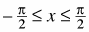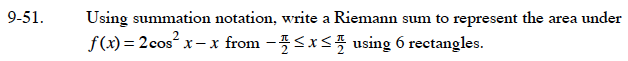### Home > CALC > Chapter 9 > Lesson 9.2.1 > Problem9-51

9-51.

Using summation notation, write a Riemann sum to represent the area under f(x) = 2cos2 xx fromusing 6 rectangles. Homework Help ✎The length of the interval is π. Using 6 rectangles, what is the width of each rectangle?